# 36 Results

View
Selected filters:
• Inequalities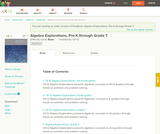Rating

CK-12 Algebra Explorations is a hands-on series of activities that guides students from Pre-K to Grade 7 through algebraic concepts.

Subject:
Algebra
Functions
Material Type:
Activity/Lab
Lesson Plan
Textbook
Provider:
CK-12 Foundation
Provider Set:
CK-12 FlexBook
Author:
Mary Cavanagh, Carol Findell, Carole Greenes
10/04/2011Conditions of Use:
Remix and Share
Rating

This site teaches Reasoning with Equations and Inequalities to High Schoolers through a series of 5909 questions and interactive activities aligned to 36 Common Core mathematics skills.

Subject:
Mathematics
Algebra
Material Type:
Activity/Lab
Interactive
Provider:
Provider Set:
01/09/2015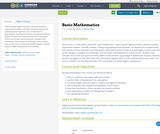Conditions of Use:
No Strings Attached
Rating

Topics include signed numbers, decimal numbers, exponential notation, scientific notation, solving and graphing linear equations, an introduction to polynomials, and systems of linear equations and their graphs. Geometrical topics include lines and angles, closed curves and convex polygons, triangles and similarities, and symmetry and proportion in nature and art. All course content by John McColgan. Content added to OER Commons by Victoria Vidal.

Subject:
Mathematics
Numbers and Operations
Material Type:
Syllabus
Author:
Victoria Vidal
10/10/2019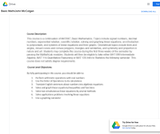Conditions of Use:
No Strings Attached
Rating

This course is a continuation of MAT087, Basic Mathematics. Topics include signed numbers, decimal numbers, exponential notation, scientific notation, solving and graphing linear equations, an introduction to polynomials, and systems of linear equations and their graphs. Geometrical topics include lines and angles, closed curves and convex polygons, triangles and similarities, and symmetry and proportion in nature and art. Students may complete this course during the first three weeks of the semester by passing the MyMathLab modules. Students will then be eligible to take either MAT 099 Intermediate Algebra, MAT 114-Quantitative Reasoning or MAT 120-Intro to Statistics the following semester. This course does not satisfy degree requirements.

Subject:
Mathematics
Material Type:
Full Course
Provider:
Roxbury Community College
Author:
John McColgan
05/15/2019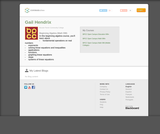Rating

In this beginning algebra course, you'll learn about
fundamental operations on real numbers
exponents
solving linear equations and inequalities
applications
functions
graphing linear equations
slope
systems of linear equations

Subject:
Algebra
Material Type:
Full Course
Lecture
Lesson
Module
Provider:
Bossier Parish Community College
Author:
Gail Hendrix
10/16/2017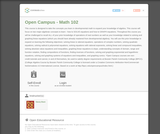Rating

This course is designed to take the concepts you learn in developmental math to expand your knowledge of algebra. This course will focus on two major algebraic concepts to learn - how to SOLVE equations and how to GRAPH equations. Throughout this course you will be challenged to recall ALL of your prior knowledge of operations of real numbers as well as your knowledge related to solving and graphing linear equations (which you should have already mastered from developmental algebra). You will use this prior knowledge to expand on learning the following objectives: solving linear & rational equations. operations of complex numbers, solving quadratic equations, solving radical & polynomial equations, solving equations with rational exponents, solving linear and compound inequalities, solving absolute value equations and inequalities, graphing linear equations & slope, understanding concepts of domain, range and function notation, finding compositions of functions, finding inverses of functions, solving and graphing exponential and logarithmic equations, solving and graphing systems of equations and inequalities, and graphing conics.

*Open Campus courses are non-credit tutorials and cannot, in and of themselves, be used to satisfy degree requirements at Bossier Parish Community College (BPCC). (College Algebra Course by Bossier Parish Community College is licensed under a Creative Commons Attribution-NonCommercial-NoDerivatives 4.0 International License. Based on a work at http://bpcc.edu/opencampus/index.html.)

Subject:
Mathematics
Algebra
Material Type:
Full Course
Provider:
Bossier Parish Community College
Author:
Stacey Black
10/11/2017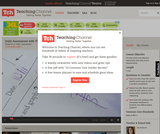Rating

Marie Barchi models an effective process of formative assessment with her Algebra I students. During the lesson, Ms. Barchi assesses student readiness as they work problems so she can identify the type of exit card appropriate for each student based on where they are at in learning how to solve inequalities.She then quickly sorts the cards to identify which students are struggling and need additional support as well as which students provided average and above average work. As students enter the classroom, she directs them to one of three groups based on the information she gathered from their exit card assessment. This grouping allows for efficient and effective differentiation.

Subject:
Algebra
Material Type:
Lesson Plan
Teaching/Learning Strategy
Provider:
Teaching Channel
Provider Set:
Teaching Channel
02/26/2013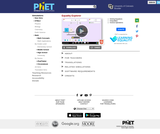Conditions of Use:
No Strings Attached
Rating

Explore what it means for a mathematical statement to be balanced or unbalanced by interacting with objects on a balance. Discover the rules for keeping it balanced. Collect stars by playing the game!

Subject:
Mathematics
Material Type:
Simulation
Provider:
Provider Set:
PhET Interactive Simulations
Author:
David Wood
Marleene Buttice
Meenakshi Raghavan
03/06/2019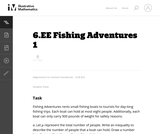Conditions of Use:
No Strings Attached
Rating

This task is the first in a series of three tasks that use inequalities in the same context at increasing complexity in 6th grade, 7th grade and in HS algebra. Students write and solve inequalities, and represent the solutions graphically.

Subject:
Mathematics
Material Type:
Activity/Lab
Provider:
Illustrative Mathematics
Provider Set:
Illustrative Mathematics
Author:
Illustrative Mathematics
05/01/2012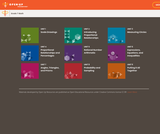Conditions of Use:
No Strings Attached
Rating

Student-facing 7th grade math resources. Covers scale drawings, proportion, percentages, probability, expressions, and geometry.

Subject:
Mathematics
Material Type:
Unit of Study
Provider:
Open Up Resources
05/21/2019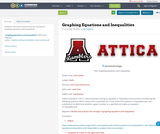Conditions of Use:
Remix and Share
Rating

Students will review and practice the concepts of graphing equations and inequalities

Subject:
Mathematics
Material Type:
Activity/Lab
Author:
Joe Ferguson
07/10/2017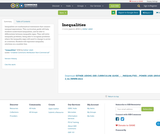Conditions of Use:
Remix and Share
Rating

Inequalities are mathematical statements that connect unequal expressions.
This curriculum guide will help students understand inequalities, and be able to differentiate between inequality signs. They will solve inequality problems, being able to recognize problems where the inequality signs will need to change to arrive at a solution. Students will represent inequality solutions on a number line.

Subject:
Mathematics
Material Type:
Lecture Notes
Lesson Plan
Author:
Esther Udoh
06/06/2018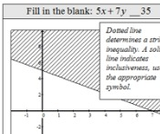Conditions of Use:
Remix and Share
Rating

This seminar will show you how to graph a linear inequality in two variables on a Cartesian Coordinate Plane. You will learn when and why to use dashed lines and solid lines. You will also learn why it is necessary to shade a half-plane, as well as how to determine which half-plane to shade.StandardsCC.2.2.HS.D.10Represent, solve, and interpret equations/inequalities and systems of equations/inequalities algebraically and graphically.

Subject:
Mathematics
Algebra
Material Type:
Lesson Plan
Author:
Bonnie Waltz
10/15/2017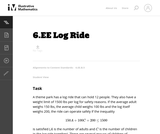Conditions of Use:
No Strings Attached
Rating

In this instructional task students are given two inequalities, one as a formula and one in words, and a set of possible solutions. They have to decide which of the given numbers actually solve the inequalities.

Subject:
Mathematics
Material Type:
Activity/Lab
Provider:
Illustrative Mathematics
Provider Set:
Illustrative Mathematics
Author:
Illustrative Mathematics
05/01/2012Conditions of Use:
Remix and Share
Rating

Subject:
Mathematics
Material Type:
Full Course
Provider:
Pearson
10/06/2016Conditions of Use:
Remix and Share
Rating

Equations and Inequalities

Type of Unit: Concept

Prior Knowledge

Students should be able to:

Add, subtract, multiply, and divide with whole numbers, fractions, and decimals.
Use the symbols <, >, and =.
Evaluate expressions for specific values of their variables.
Identify when two expressions are equivalent.
Simplify expressions using the distributive property and by combining like terms.
Use ratio and rate reasoning to solve real-world problems.
Order rational numbers.
Represent rational numbers on a number line.

Lesson Flow

In the exploratory lesson, students use a balance scale to find a counterfeit coin that weighs less than the genuine coins. Then continuing with a balance scale, students write mathematical equations and inequalities, identify numbers that are, or are not, solutions to an equation or an inequality, and learn how to use the addition and multiplication properties of equality to solve equations. Students then learn how to use equations to solve word problems, including word problems that can be solved by writing a proportion. Finally, students connect inequalities and their graphs to real-world situations.

Subject:
Mathematics
Algebra
Material Type:
Unit of Study
Provider:
Pearson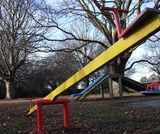Conditions of Use:
Remix and Share
Rating

Lesson OverviewUsing a balance scale, students decide whether a certain value of a variable makes a given equation or inequality true. Then students extend what they learned using the balance scale to substituting a given value for a variable into an equation or inequality to decide if that value makes the equation or inequality true or false.Key ConceptsStudents will extend what they know about substituting a value for a variable into an expression to evaluate that expression.Equations and inequalities may contain variables. These equations or inequalities are neither true nor false. When a value is substituted for a variable, the equation or inequality then becomes true or false. If the equation or inequality is true for that value of the variable, that value is considered a solution to the equation or inequality.Goals and Learning ObjectivesUnderstand what solving an equation or inequality means.Use substitution to determine whether a given number makes an equation or inequality true.

Subject:
Algebra
Material Type:
Lesson Plan
Provider:
Pearson
09/21/2015Conditions of Use:
Remix and Share
Rating

Lesson OverviewStudents use weights to represent equal and unequal situations on a balance scale and represent them symbolically.Key ConceptsAn equation is a statement that shows that two expressions are equivalent. An equal sign (=) is used between the two expressions to indicate that they are equivalent. You can think of the two expressions as being “balanced.”An inequality is a statement that shows that two expressions are unequal. The symbols for “greater than” (>) and “less than” (<) are used to indicate which expression has the greater or lesser value. In an inequality, you can think of the two expressions as being “unbalanced.”Goals and Learning ObjectivesExplore a balance scale as a model for equations and inequalities.Understand that an equation states that two expressions are equivalent using an equal sign (=).Understand that an inequality states that one expression is greater than (>) or is less than (<) another expression.Use the equal sign (=) and the greater than (>) and less than (<) symbols with rational numbers.

Subject:
Algebra
Material Type:
Lesson Plan
Provider:
Pearson
09/21/2015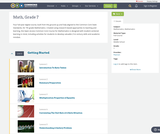Conditions of Use:
Remix and Share
Rating

Four full-year digital course, built from the ground up and fully-aligned to the Common Core State Standards, for 7th grade Mathematics. Created using research-based approaches to teaching and learning, the Open Access Common Core Course for Mathematics is designed with student-centered learning in mind, including activities for students to develop valuable 21st century skills and academic mindset.

Subject:
Mathematics
Material Type:
Full Course
Provider:
Pearson
10/06/2016Conditions of Use:
Remix and Share
Rating

Algebraic Reasoning

Type of Unit: Concept

Prior Knowledge

Students should be able to:

Add, subtract, multiply, and divide rational numbers.
Evaluate expressions for a value of a variable.
Use the distributive property to generate equivalent expressions including combining like terms.
Understand solving an equation or inequality as a process of answering a question: which values from a specified set, if any, make the equation or inequality true?
Write and solve equations of the form x+p=q and px=q for cases in which p, q, and x are non-negative rational numbers.
Understand and graph solutions to inequalities x<c or x>c.
Use equations, tables, and graphs to represent the relationship between two variables.
Relate fractions, decimals, and percents.
Solve percent problems included those involving percent of increase or percent of decrease.

Lesson Flow

This unit covers all of the Common Core State Standards for Expressions and Equations in Grade 7. Students extend what they learned in Grade 6 about evaluating expressions and using properties to write equivalent expressions. They write, evaluate, and simplify expressions that now contain both positive and negative rational numbers. They write algebraic expressions for problem situations and discuss how different equivalent expressions can be used to represent different ways of solving the same problem. They make connections between various forms of rational numbers. Students apply what they learned in Grade 6 about solving equations such as x+2=6 or 3x=12 to solving equations such as 3x+6=12 and 3(x−2)=12. Students solve these equations using formal algebraic methods. The numbers in these equations can now be rational numbers. They use estimation and mental math to estimate solutions. They learn how solving linear inequalities differs from solving linear equations and then they solve and graph linear inequalities such as −3x+4<12. Students use inequalities to solve real-world problems, solving the problem first by arithmetic and then by writing and solving an inequality. They see that the solution of the algebraic inequality may differ from the solution to the problem.

Subject:
Mathematics
Algebra
Material Type:
Unit of Study
Provider:
Pearson